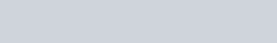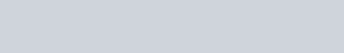Two charges
Question:

Two charges $5 \times 10^{-8} \mathrm{C}$ and $-3 \times 10^{-8} \mathrm{C}$ are located $16 \mathrm{~cm}$ apart. At what point(s) on the line joining the two charges is the electric potential zero? Take the potential at infinity to be zero.

Solution:

There are two charges,

$q_{1}=5 \times 10^{-8} \mathrm{C}$

$q_{2}=-3 \times 10^{-8} \mathrm{C}$

Distance between the two charges, d = 16 cm = 0.16 m

Consider a point P on the line joining the two charges, as shown in the given figure.r = Distance of point P from charge q1

Let the electric potential (V) at point P be zero.

Potential at point P is the sum of potentials caused by charges q1 and q2 respectively.

$\therefore V=\frac{q_{1}}{4 \pi \epsilon_{0} r}+\frac{q_{2}}{4 \pi \in_{0}(d-r)}$    …(i)

Where,

$\epsilon_{0}=$ Permittivity of free space

For V = 0, equation (i) reduces to

$\frac{q_{1}}{4 \pi \in_{0} r}=-\frac{q_{2}}{4 \pi \in_{0}(d-r)}$

$\frac{q_{1}}{r}=\frac{-q_{2}}{d-r}$

$\frac{5 \times 10^{-8}}{r}=-\frac{\left(-3 \times 10^{-8}\right)}{(0.16-r)}$

$\frac{0.16}{r}-1=\frac{3}{5}$

$\frac{0.16}{r}=\frac{8}{5}$

$\therefore r=0.1 \mathrm{~m}=10 \mathrm{~cm}$

Therefore, the potential is zero at a distance of 10 cm from the positive charge between the charges.

Suppose point P is outside the system of two charges at a distance from the negative charge, where potential is zero, as shown in the following figure.For this arrangement, potential is given by,

$V=\frac{q_{1}}{4 \pi \in_{0} s}+\frac{q_{2}}{4 \pi \in_{0}(s-d)}$  …(ii)

For $V=0$, equation (ii) reduces to

$\frac{q_{1}}{4 \pi \epsilon_{0} s}=-\frac{q_{2}}{4 \pi \in_{0}(s-d)}$

$\frac{q_{1}}{s}=\frac{-q_{2}}{s-d}$

$\frac{5 \times 10^{-8}}{s}=-\frac{\left(-3 \times 10^{-8}\right)}{(s-0.16)}$

$1-\frac{0.16}{s}=\frac{3}{5}$

$\frac{0.16}{s}=\frac{2}{5}$

$\therefore s=0.4 \mathrm{~m}=40 \mathrm{~cm}$

Therefore, the potential is zero at a distance of 40 cm from the positive charge outside the system of charges.